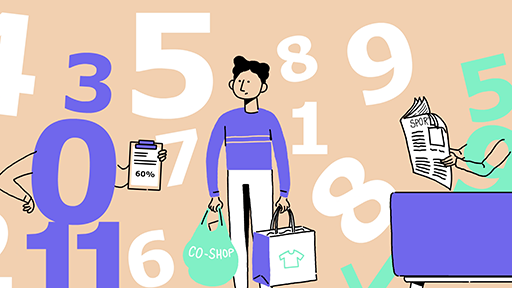Science, Maths & Technology

### Become an OU studentEveryday maths 1 (Wales)

Start this free course now. Just create an account and sign in. Enrol and complete the course for a free statement of participation or digital badge if available.

# Session 1: Working with numbers

## Introduction

It is very difficult to cope in everyday life without a basic understanding of numbers.

Calculators can be very useful, for example helping you to check your working out, or converting fractions to decimals.

To complete the activities in this course you will need some notepaper, a pen for taking notes and working out calculations and a calculator.

Session 1 includes many examples of numeracy from everyday life, with lots of learning activities related to them that involve whole numbers, fractions, decimals, percentages, ratios and proportion.

By the end of this session you will be able to:

• work with whole numbers
• use rounding
• understand fractions, decimals and percentages, and the equivalencies between them
• use ratios and proportion
• understand word formulas and function machines.